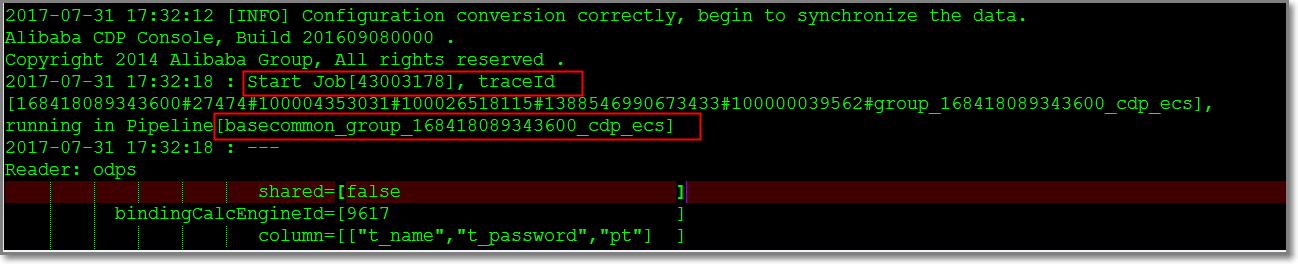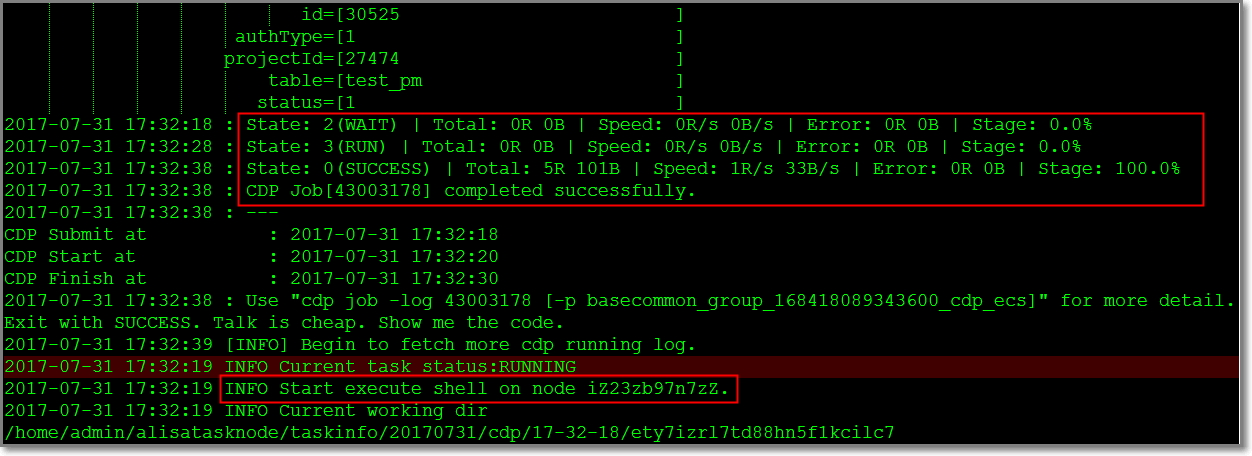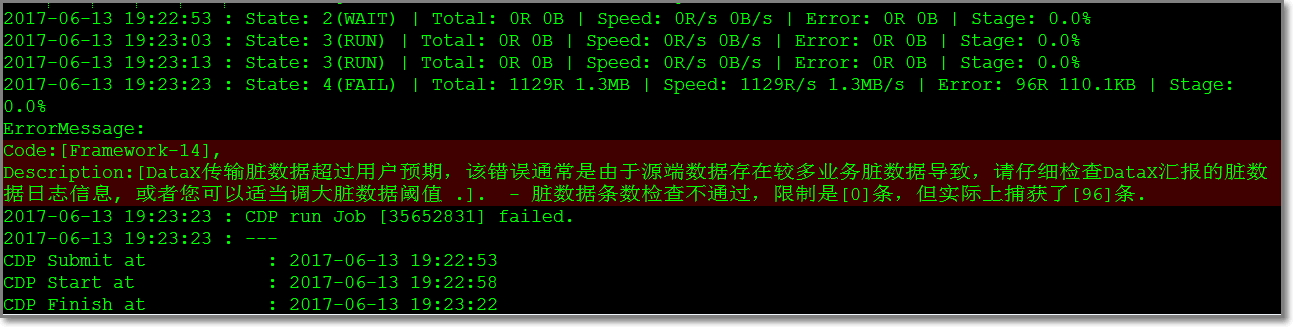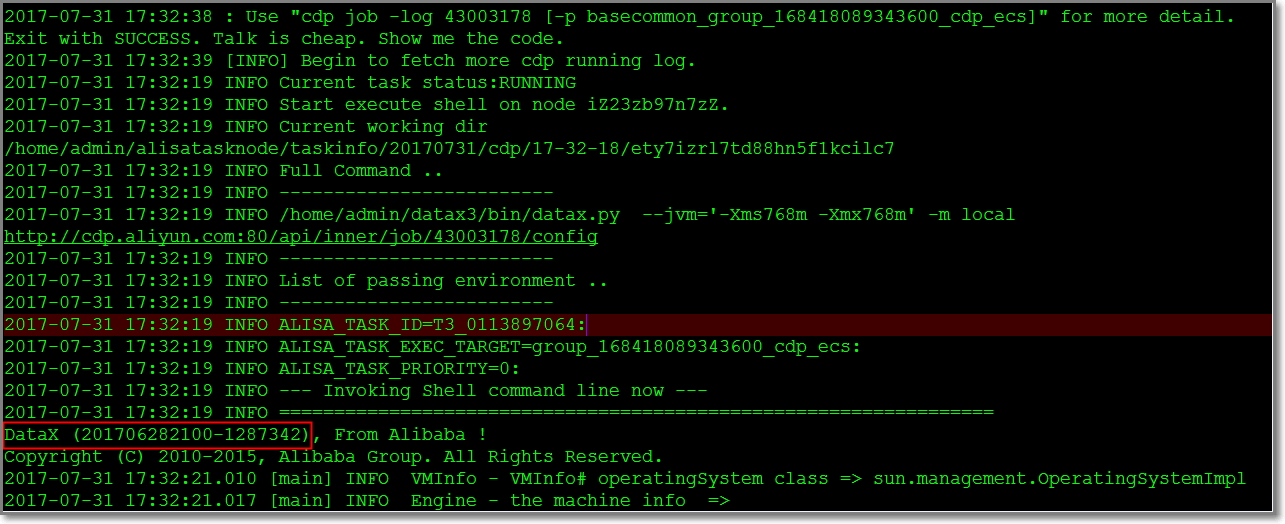## 步骤4：查看同步日志

### 任务是从哪里开始的### 实际运行的任务代码

1. `Reader: odps `
2. ` shared=[false ]`
3. ` bindingCalcEngineId=[9617 ]`
4. ` column=[["t_name","t_password","pt"] ]`
5. ` description=[connection from odps calc engine 9617]`
6. ` project=[XXXXXXXXX ]`
7. ` *accessKey=[******** ]`
8. ` gmtCreate=[2016-10-13 16:42:19 ]`
9. ` type=[odps ]`
10. ` accessId=[XXXXXXXXX ]`
11. ` datasourceType=[odps ]`
12. ` odpsServer=[http://service.odps.aliyun.com/api]`
13. ` endpoint=[http://service.odps.aliyun.com/api]`
14. ` partition=[pt=20170425 ]`
15. ` datasourceBackUp=[odps_first ]`
16. ` name=[odps_first ]`
17. ` tenantId=[168418089343600 ]`
18. ` subType=[ ]`
19. ` id=[30525 ]`
20. ` authType=[1 ]`
21. ` projectId=[27474 ]`
22. ` table=[t_name ]`
23. ` status=[1 ]`
24. `Writer: odps `
25. ` shared=[false ]`
26. ` bindingCalcEngineId=[9617 ]`
27. ` column=[["id","name","pt"] ]`
28. ` description=[connection from odps calc engine 9617]`
29. ` project=[XXXXXXXXX ]`
30. ` *accessKey=[******** ]`
31. ` gmtCreate=[2016-10-13 16:42:19 ]`
32. ` type=[odps ]`
33. ` accessId=[XXXXXXXXX ]`
34. ` datasourceType=[odps ]`
35. ` odpsServer=[http://service.odps.aliyun.com/api]`
36. ` endpoint=[http://service.odps.aliyun.com/api]`
37. ` partition=[ ]`
38. ` truncate=[true ]`
39. ` datasourceBackUp=[odps_first ]`
40. ` name=[odps_first ]`
41. ` tenantId=[XXXXXXXXX ]`
42. ` subType=[ ]`
43. ` id=[30525 ]`
44. ` authType=[1 ]`
45. ` projectId=[27474 ]`
46. ` table=[test_pm ]`
47. ` status=[1 ]`

### 任务运行情况### 详细运行日志